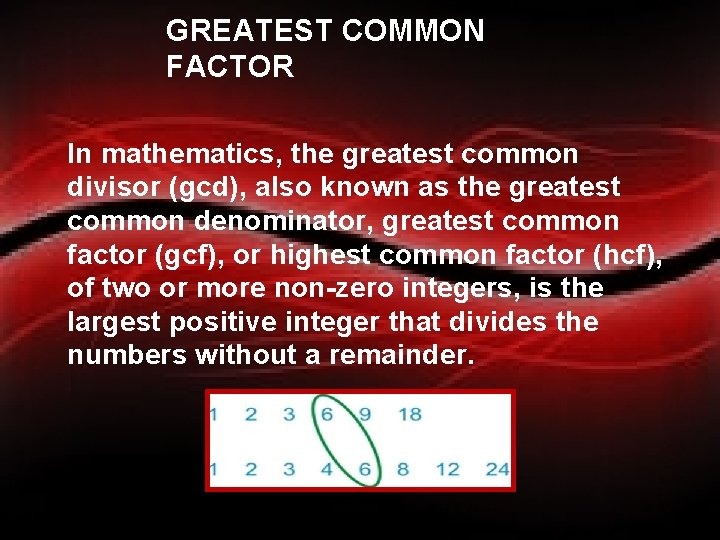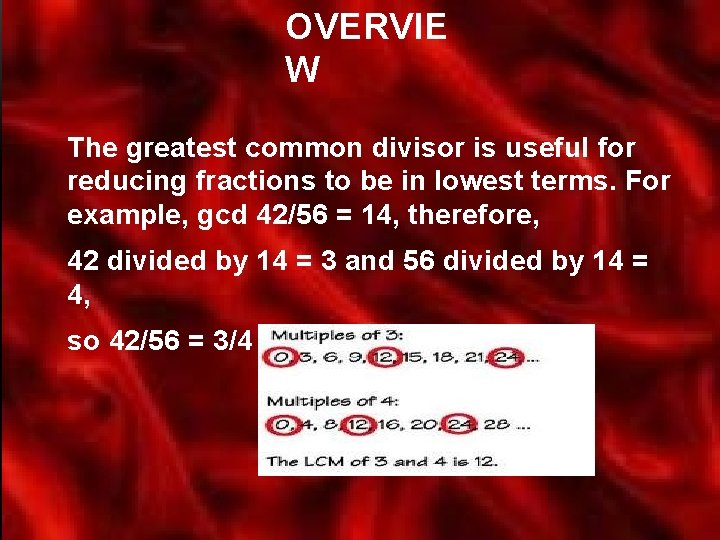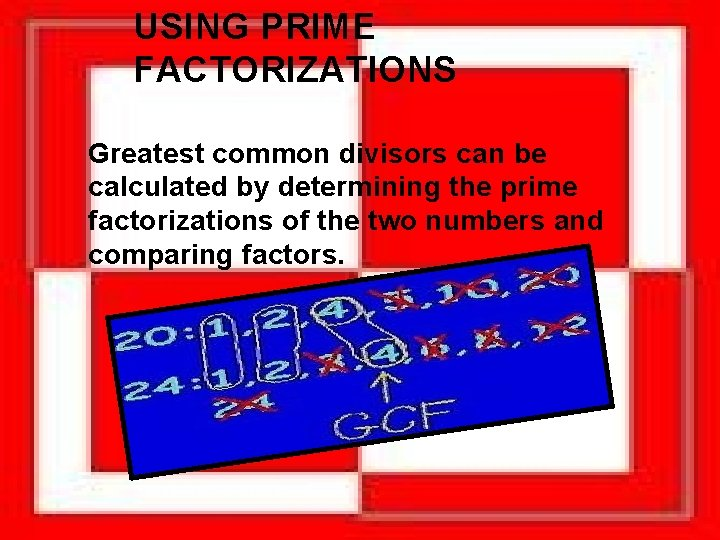# GREATEST COMMON FACTOR Eduardo Lira GREATEST COMMON FACTOR

• Slides: 10GREATEST COMMON FACTOR Eduardo LiraGREATEST COMMON FACTOR In mathematics, the greatest common divisor (gcd), also known as the greatest common denominator, greatest common factor (gcf), or highest common factor (hcf), of two or more non-zero integers, is the largest positive integer that divides the numbers without a remainder.OVERVIE W The greatest common divisor is useful for reducing fractions to be in lowest terms. For example, gcd 42/56 = 14, therefore, 42 divided by 14 = 3 and 56 divided by 14 = 4, so 42/56 = 3/4USING PRIME FACTORIZATIONS Greatest common divisors can be calculated by determining the prime factorizations of the two numbers and comparing factors.EXAMPL E To calculate the gcd of 18/84, we find the prime factorizations: 18 = 1, 2, 3, 6, 9, 18 and 84 = 1, 2, 3, 4, 6, 7, 12, 14, 16, 21, 28, 42, 84, and notice that the "overlap" of the gcf is 6, so the gcd of 18/84 = 6.EXAMPL E Factors are the numbers you multiply together to get another number: . Sometimes we want to find all the factors of a number: the factors of 12 are 1, 2, 3, 4, 6 and 12. . . because 2 × 6 = 12, or 4 × 3 = 12, or 1 × 12 = 12.The greatest common factor, or GCF, is the greatest factor that divides two numbers. To find the GCF of two numbers: List the prime factors of each number. Multiply those factors both numbers have in common. If there are no common prime factors, the GCF is 1.QUESTION What is the greatest common factor of 15 and 5? The answer is 5 because 15 = 3 x 5 and 5 = 1 x 5.GREATEST COMMON FACTOR The Greatest Common Factor (GCF) is the largest number that is a common factor of two or more numbers. How to find the greatest common factor: Determine if there is a common factor of the numbers. A common factor is a number that will divide into both numbers evenly. Two is a common factor of 4 and 14. Divide all of the numbers by this common factor. Repeat this process with the resulting numbers until there are no more common factors. Multiply all of the common factors together to find the Greatest Common Factor.